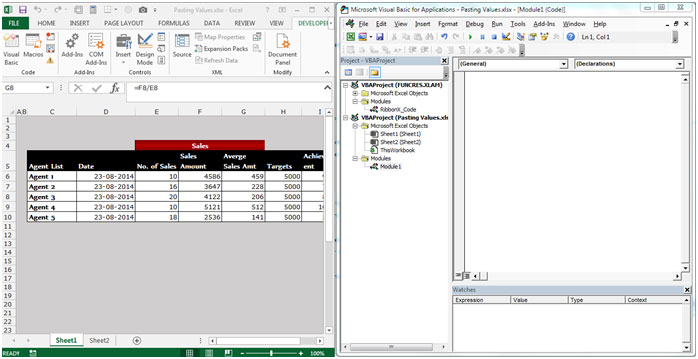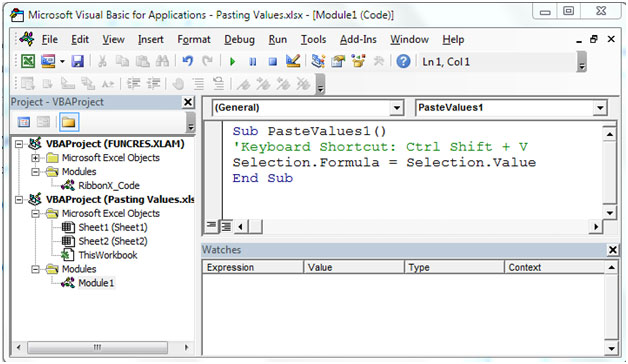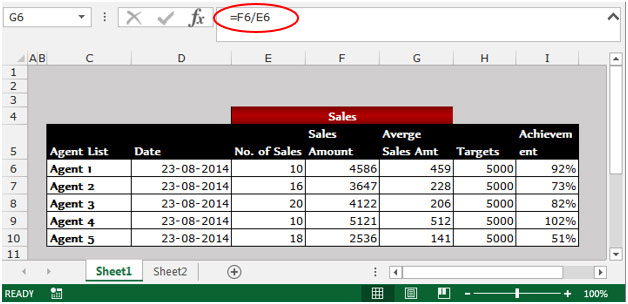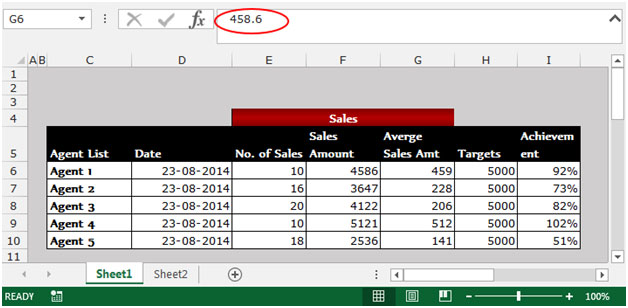Pasting Values in Microsoft Excel 2010

In this article, we will learn how to paste the cell values by using the Paste Special function or how we can write the VBA code in Microsoft Excel 2010.

Paste Special: You can use the Paste Special to paste the specific cell content or attributes such as formula, formatting, comments etc.

To paste the calculated value of a formula into a single cell (thus overwriting the formula):- Press F2 to edit and then F9 to calculate.

To paste the calculated values from formula in a range of cells, create and add a keyboard shortcut using the VBE.

To write the code in VBA, follow below given steps:-

• Press the key Alt+F11 to open the VBE.• Write the code for Paste value the formulas in VBE.Sub PasteValues ()

‘ Keyboard Shortcut: Ctrl+Shift+V

Selection.Formula=Selection.Value

End Sub

• To run the Macro, press the key F5.
• Selected cell will convert into Paste Special>Value.

Image Before to Run the MacroImage After to Run the MacroTo paste values in a range of cells, use the Paste Special dialog box:

• Copy a range of cells that contain formulas.
• Press Shift+F10 or right click with the mouse, and then select Paste Special.• Paste Special dialog box will appear.
• Select the Value option.
• Click on OK.

This is the way we can convert the formula into values by using the Paste Special option and can write the VBE code in Microsoft Excel.

1.how to do paste in different rows at multiple times in excel?

•Hi Juned,

Could you please explain what do you mean by pasting in different rows at multiple times? Also, it would be nice if you add some example so that we can provide you the solution accordingly.

Regards,